Function Repository Resource:

# Geodesic

Compute the geodesics for a parametrized surface

Contributed by: Wolfram Staff (original content by Alfred Gray)
 ResourceFunction["Geodesic"][s,{u,v},t,{u0,v0},θ0] computes the geodesics for surface s with parameters u and v, emanating from the point parametrized by u0,v0 and proceeding in the direction θ0.

## Details and Options

A geodesic is a curve that locally minimizes length traversed.
The result returned by ResourceFunction["Geodesic"] is a set of differential equations of u and v in the variable t.
The system of equations generated by ResourceFunction["Geodesic"] has the form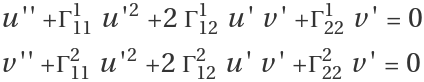, where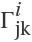are the Christoffel symbols of the second kind that can be effectively computed by the resource function ChristoffelSymbol.
Initial conditions are of the form u(0)=u0, v(0)=v0 and u'(0)=cos(θ0), v'(0)=sin(θ0).

## Examples

### Basic Examples (7)

A sphere:

 In:=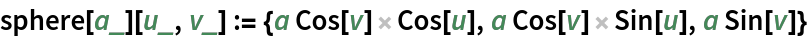Equations for geodesics on a sphere:

 In:=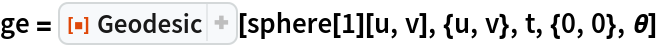Out=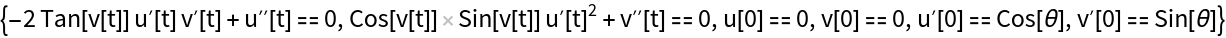A set of numerical solutions of equations for geodesics of a sphere:

 In:=Evaluate the geodesic at a definite point:

 In:=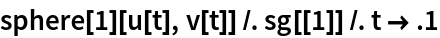Out=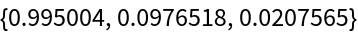Plot solutions in a plane:

 In:=Out=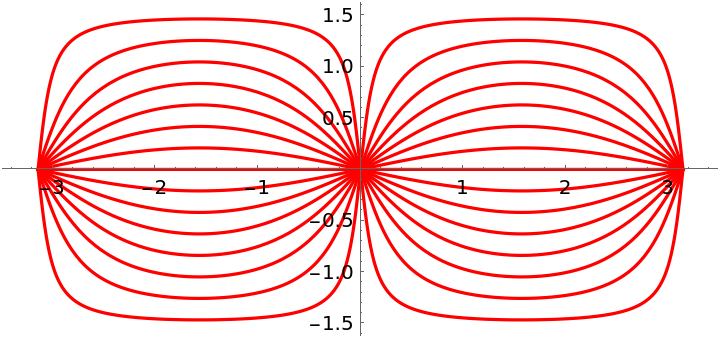Plot geodesics on a sphere:

 In:=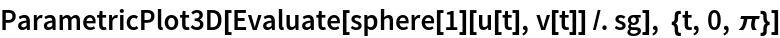Out=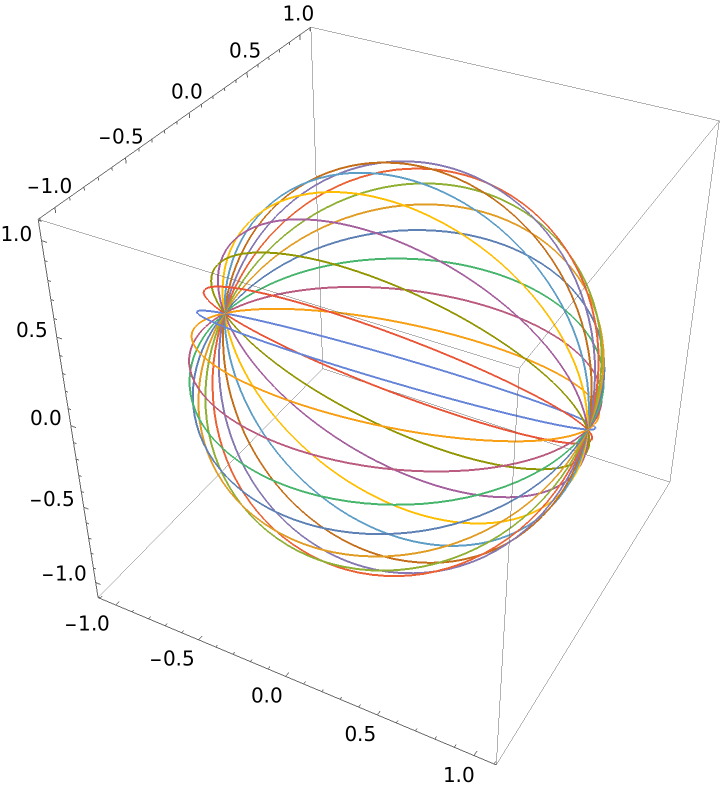Plot the geodesic circles (locus surface points located at a given geodesic radius):

 In:=Out=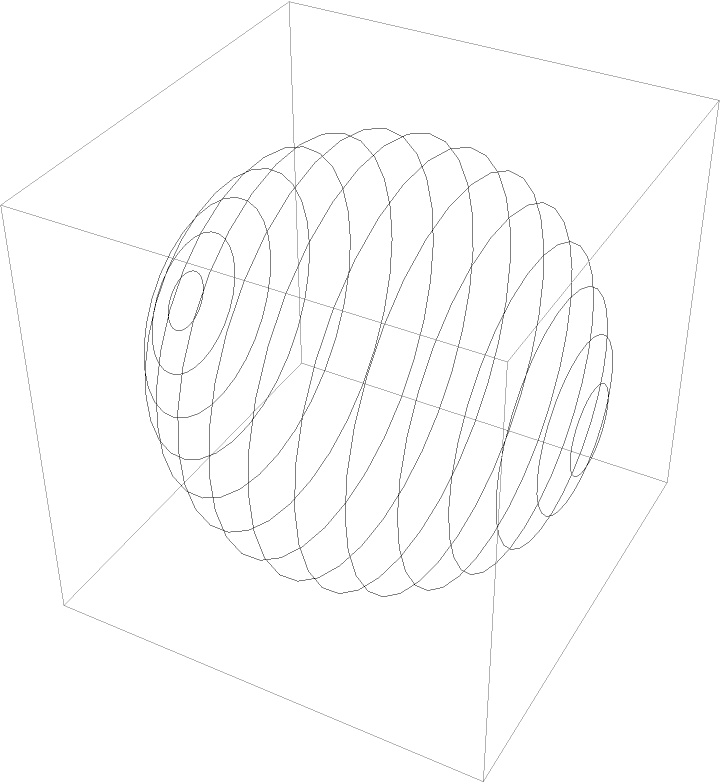### Scope (3)

A torus:

 In:=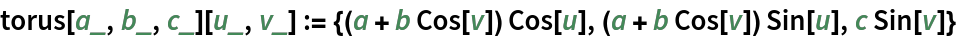The equations for geodesics:

 In:=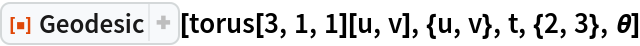Out=Solve a geodesic for large t:

 In:=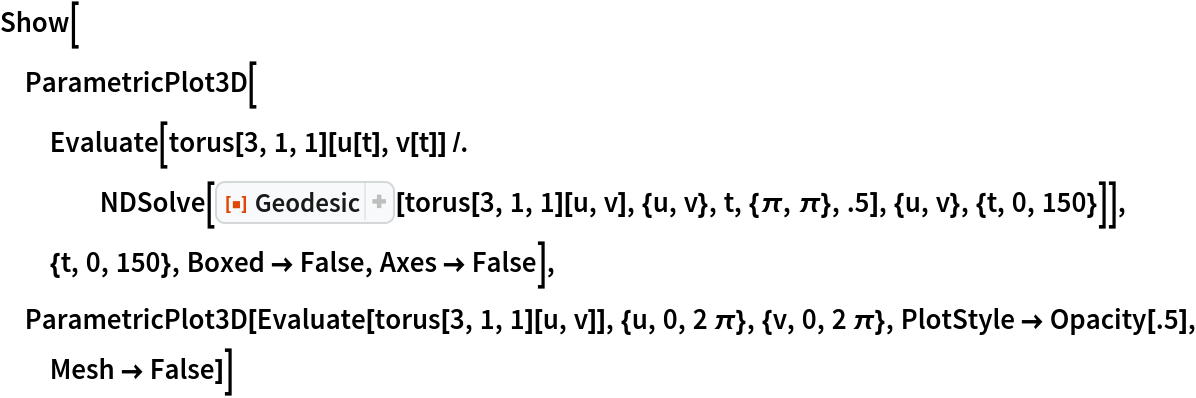Out=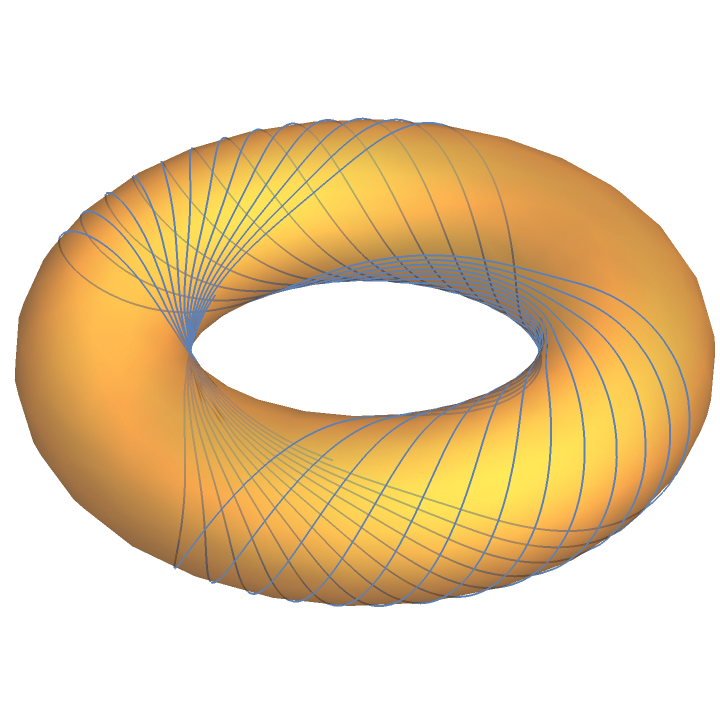Solve for geodesics:

 In:=Plot solutions in a plane:

 In:=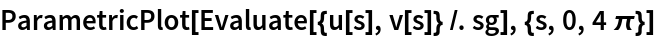Out=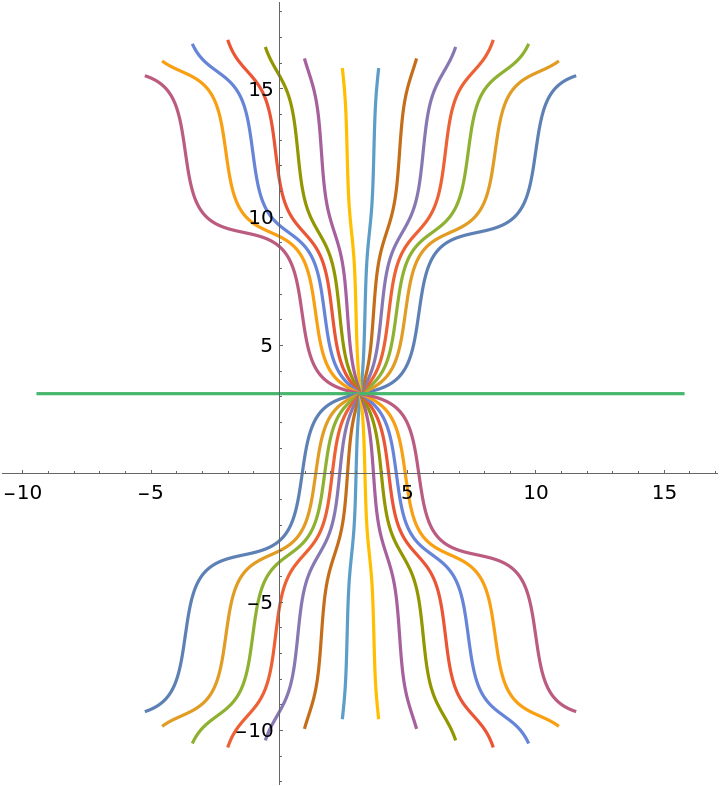Solve for a set of geodesics:

 In:=Out=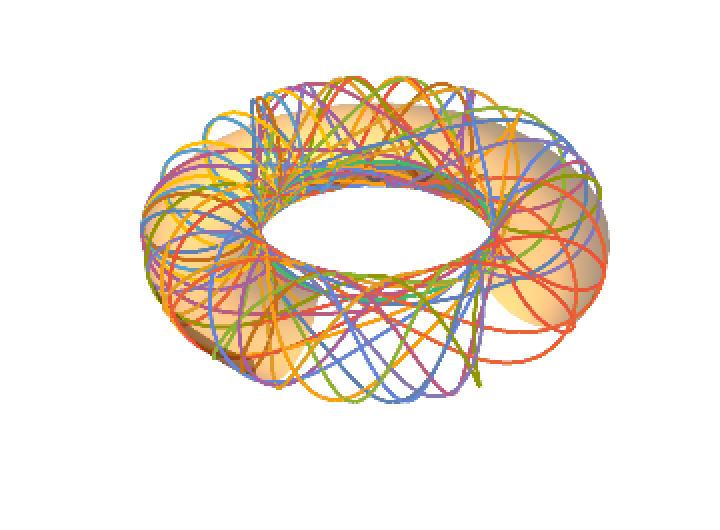Plot the geodesic circles:

 In:=Out=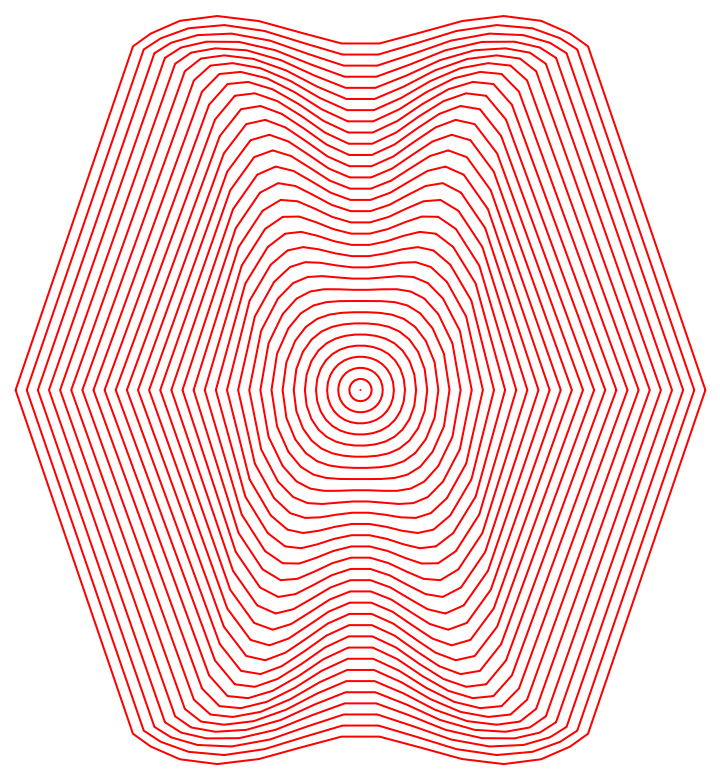Plot the geodesic circles over the torus:

 In:=In:=Out=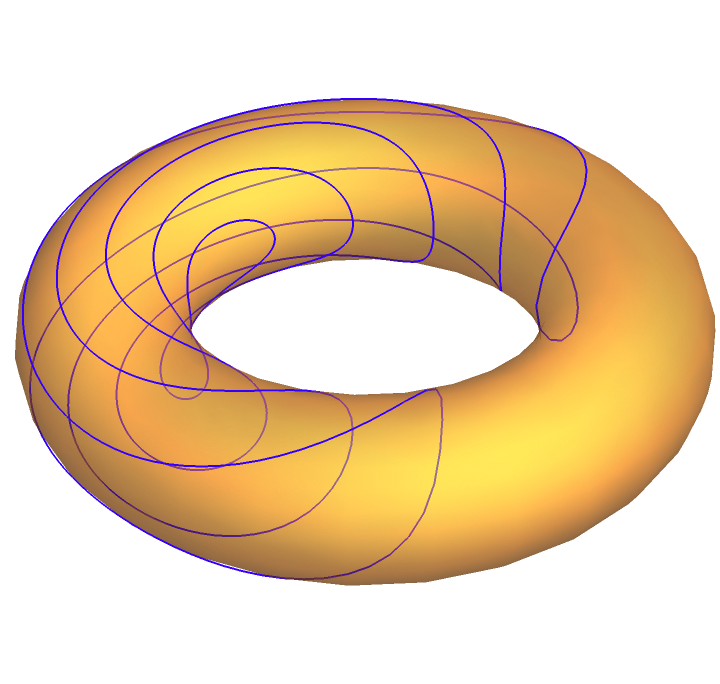In:=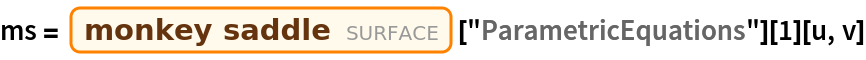Out=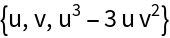Get the equations for geodesics:

 In:=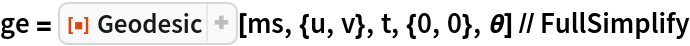Out=Solve the equations for geodesics:

 In:=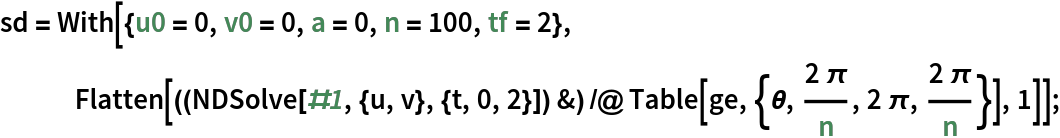Plot solutions for geodesics:

 In:=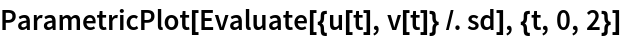Out=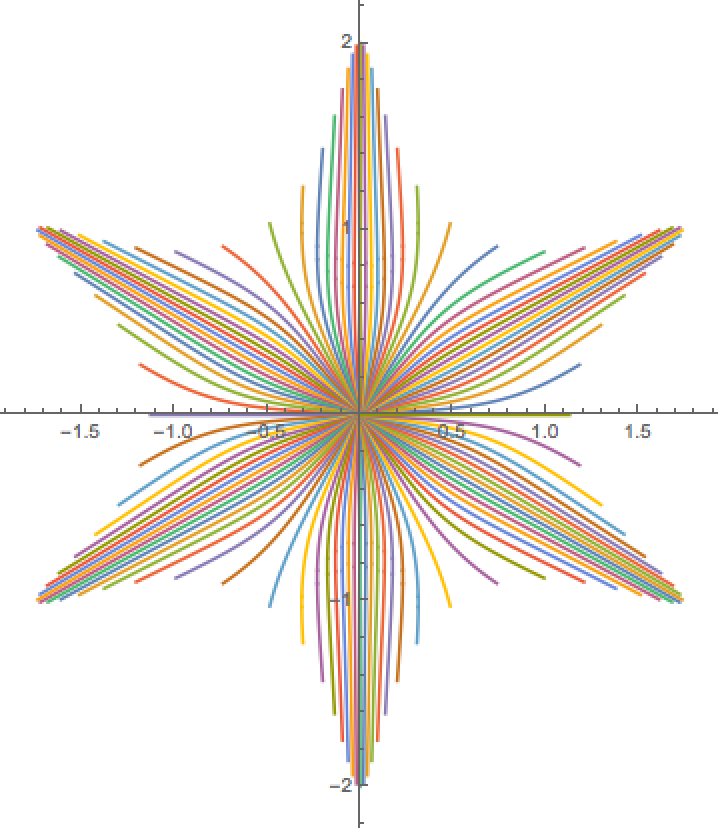Plot the geodesics over the surface:

 In:=Out=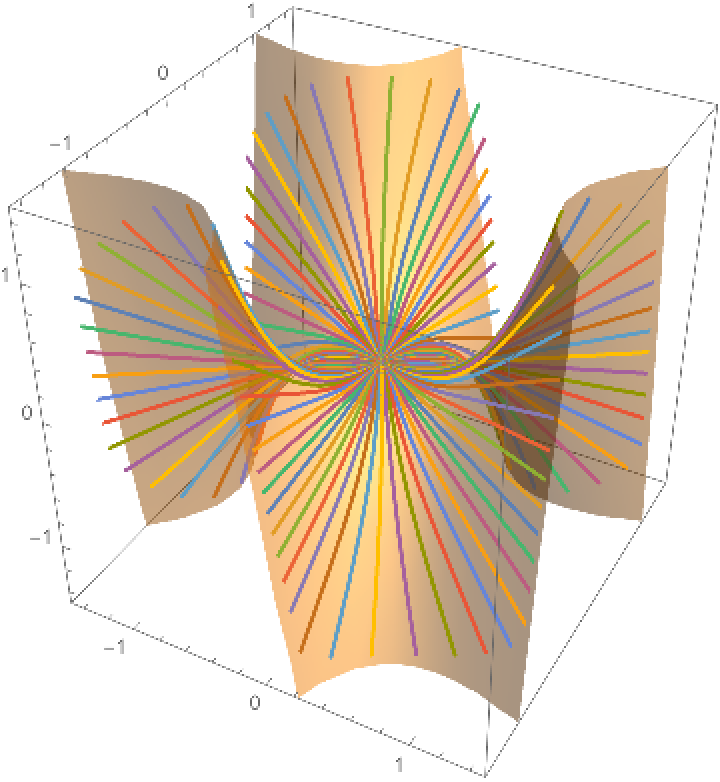Plot the geodesic circles:

 In:=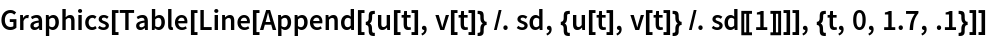Out=Plot the geodesics circles over the surface:

 In:=Out=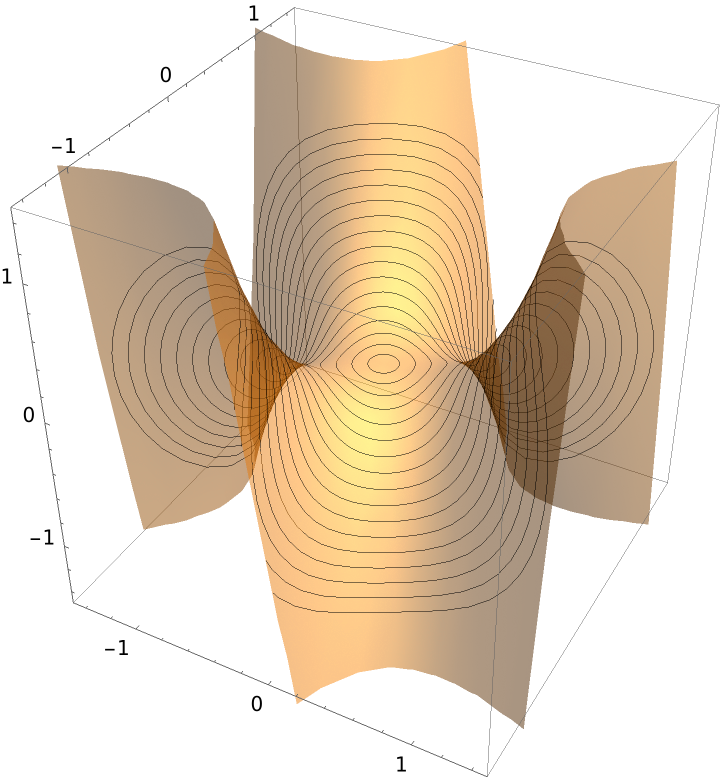A pseudosphere:

 In:=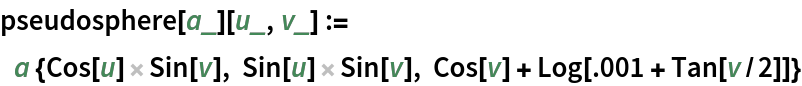The geodesic equations:

 In:=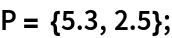In:=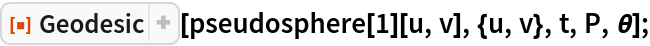Plot the geodesic equations in 3D varying θ:

 In:=In:=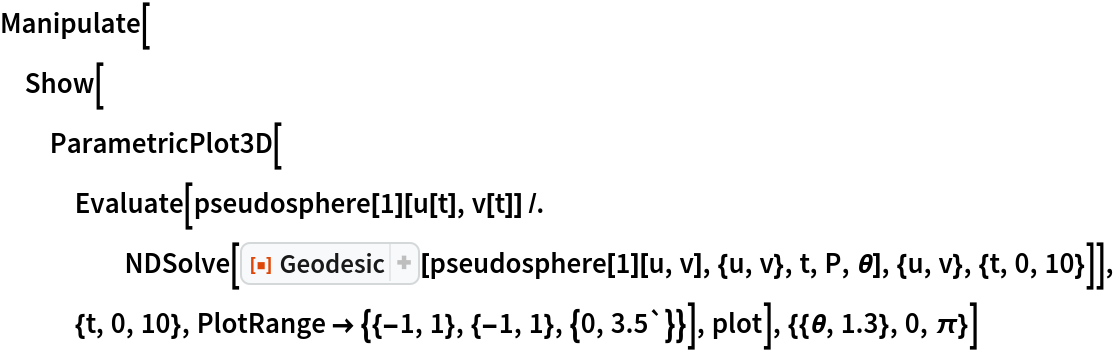Out=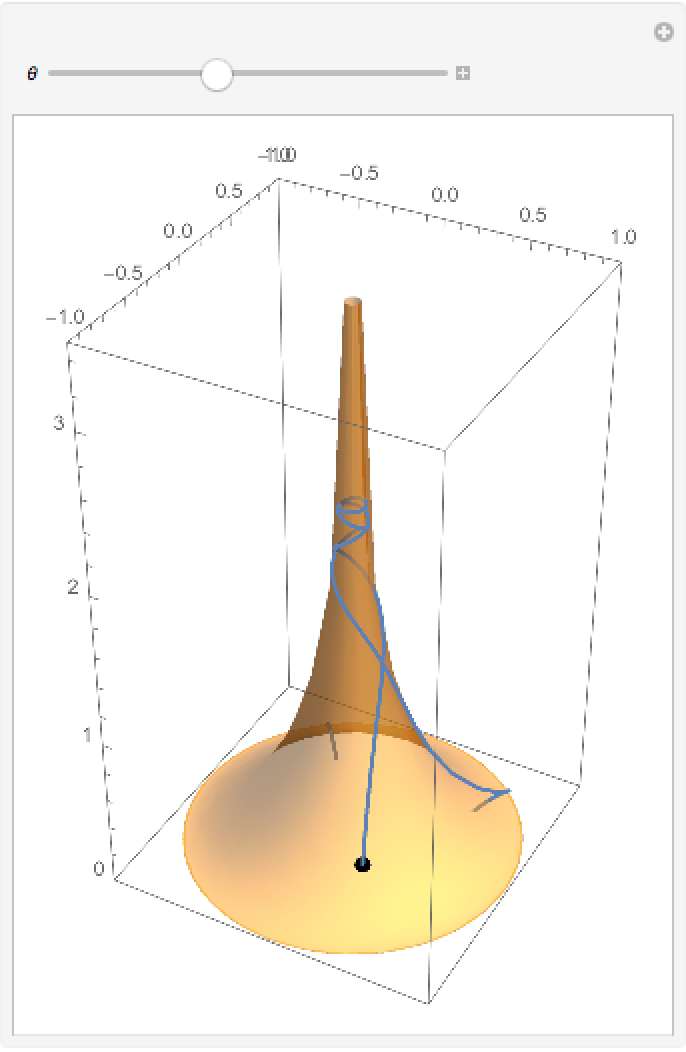A top view of a solution:

 In:=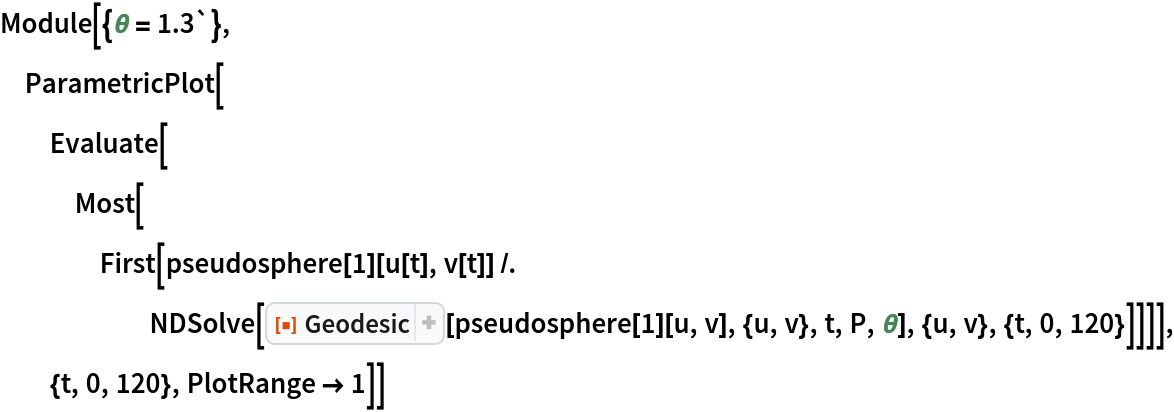Out=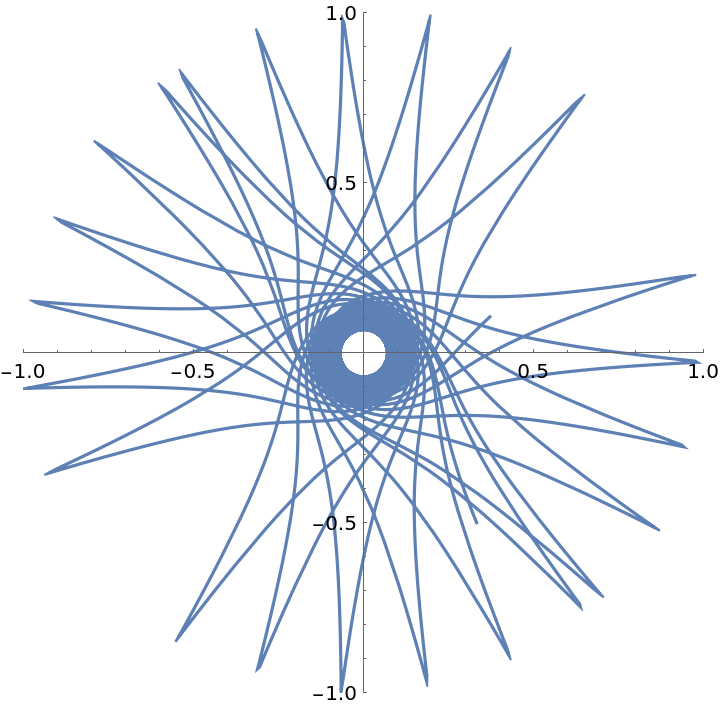Enrique Zeleny

## Version History

• 1.0.0 – 08 April 2020